Related Articles

# Properties of Limits

• Last Updated : 30 Apr, 2021

Limits form the foundation of the theory of calculus. Limits of the functions are used to define the derivatives of the functions, check the continuity in functions, and so on. Intuitively value of the limit of a function at a particular point gives us an idea about the approaching value of the function. Note that while calculating the limits we are not calculating the exact value of the function that that particular point. We are more interested in looking for the direction or to the point where the function is approaching. Let’s define limits and see properties in more detail.

### Limits

Limits are used in calculus to define differential, continuity, Integrals, and it is defined as the approaching value of the function with the input approaching to ascertain value. Let’s say we have a function f(x) = x2. In the graph given below, notice that as x⇢0, f(x) also tends to become zero. This can be written in terms of limit as,. It is read as the limit of f(x) as x tends to zero.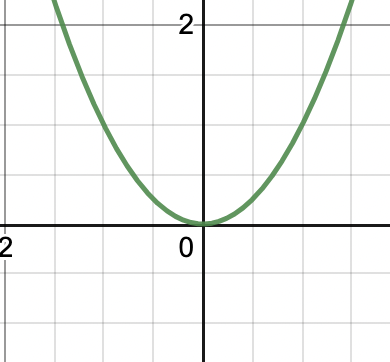In general, as x ⇢ a, f(x) ⇢ l, then l is called the limit of the function f(x). It can also be written as,Sometimes some functions are not continuous, that is they appear to be approaching two different values when they are approached from two sides. For example, let’s see this step function given in the figure below.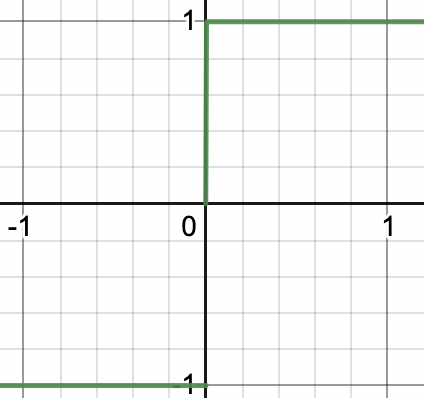This function can be defined as,Suppose we want to approach zero and see the function’s limit. This naturally leads to directions from which we can approach. Left-hand side and the right-hand side limits. The right-hand side limit is the value of the function which it takes while approaching it from the right-hand side of the desired point. Similarly, the left-hand side limit is the value of function while approaching it from the left-hand side.

For this particular function,

Left-hand side limit,Right-hand side limit,### Algebra of Limits

Let’s say we have two functions, f(x) and g(x). We know thatandexist. The properties given below describe the behavior of limits when these two functions are combined in different ways. These are presented without proof, but we will see some examples about these properties to verify them.

Property 1:

Limit of sum of two functions is the sum of limits of both the functions.Property 2:

Limit of difference of two functions is the difference of limits of both the functions.Property 3:

Limit of product of two functions is the product of limits of both the functions.Property 4:

Limit of quotient of two functions is the quotient of limits of both the functions.### Limit of Composite Functions

The composition of two functions f(x) and g(x) is denoted by (f o g)(x), which means the range of the function g(x) should lie in the domain of the function f(x). Now for calculating the limit of the composition of the two functions, we use the following property:Let’s see some sample problems on these concepts,

### Sample Problems

Question 1: Given the function f(x) =. FindSolution:

Let’s see this limit graphically,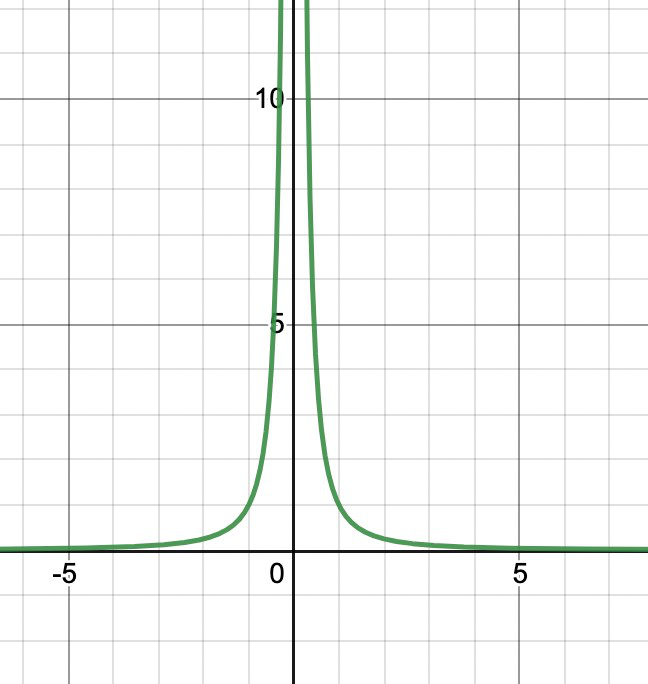We can see from the graph while approaching the function from either of the sides towards zero. Values start going to infinity.Question 2: Find the value of the limit of the function f(x) = x + cos(x) when x ⇢ 0.

Solution:

The figure below shows the graph of the function,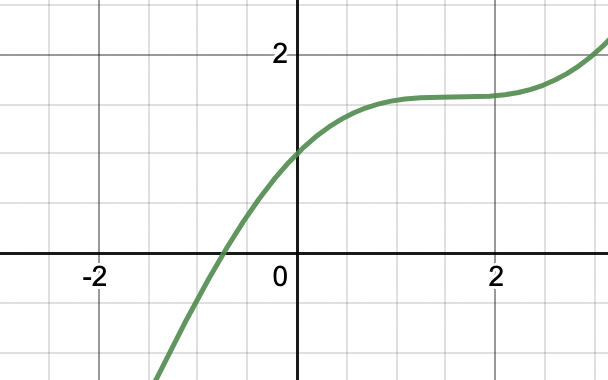We know that f(x) is a combination of two different function. We can use the properties studied above, property 1 works for our case.We know f(x) = x + cos(x). Let’s say h(x) = x and g(x) = cos(x) and using the above property we get.= 1

Question 3: Find the value of the limit of the function f(x) = (x2 + x +1)ex when x ⇢ 0.

Solution:

We know that f(x) is a combination of two different function. We can use the properties studied above, property 3 works for our case.We know f(x) = (x2 + x +1)ex Let’s say h(x) = x2 + x +1 and g(x) =ex and using the above property we get.= 1 + 1

= 2

Question 4: Find the value of the limit of the function f(x) =when x ⇢ 0.

Solution:

We know that f(x) is a combination of two different function. We can use the properties studied above, property 4 works for our case.We know f(x) =Let’s say g(x) = x2 + x +4 and g(x) =cos(x) and using the above property we get.Question 5: Find the value of the limit of the function from left-hand side and right-hand side when x ⇢ 0, f(x) =.

Solution:

Let’s see this limit graphically,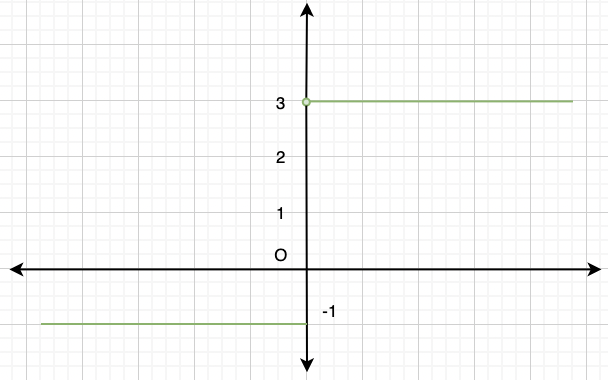Notice in the graph that while approaching from the left-hand side, the functions seems to take value -1 and while approaching from the right-hand side, functions seems to taking value 3.

Thus,Attention reader! Don’t stop learning now. Join the First-Step-to-DSA Course for Class 9 to 12 students , specifically designed to introduce data structures and algorithms to the class 9 to 12 students

My Personal Notes arrow_drop_up